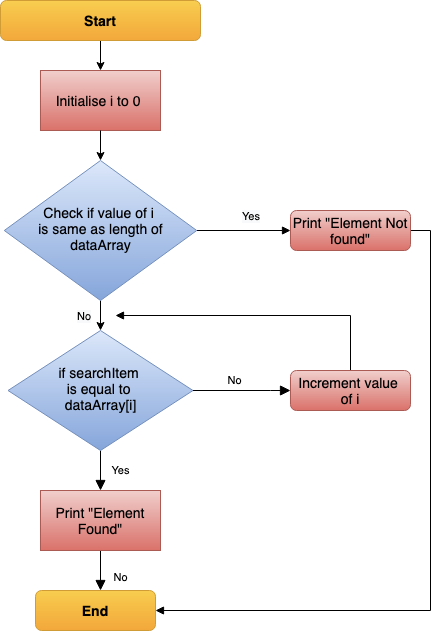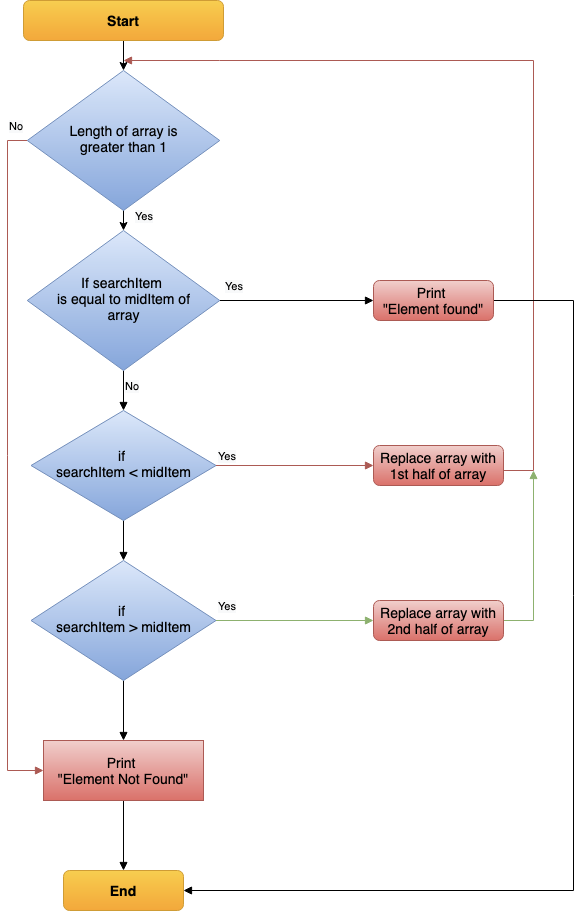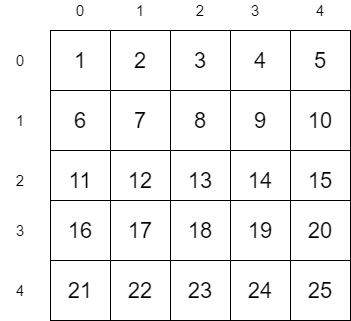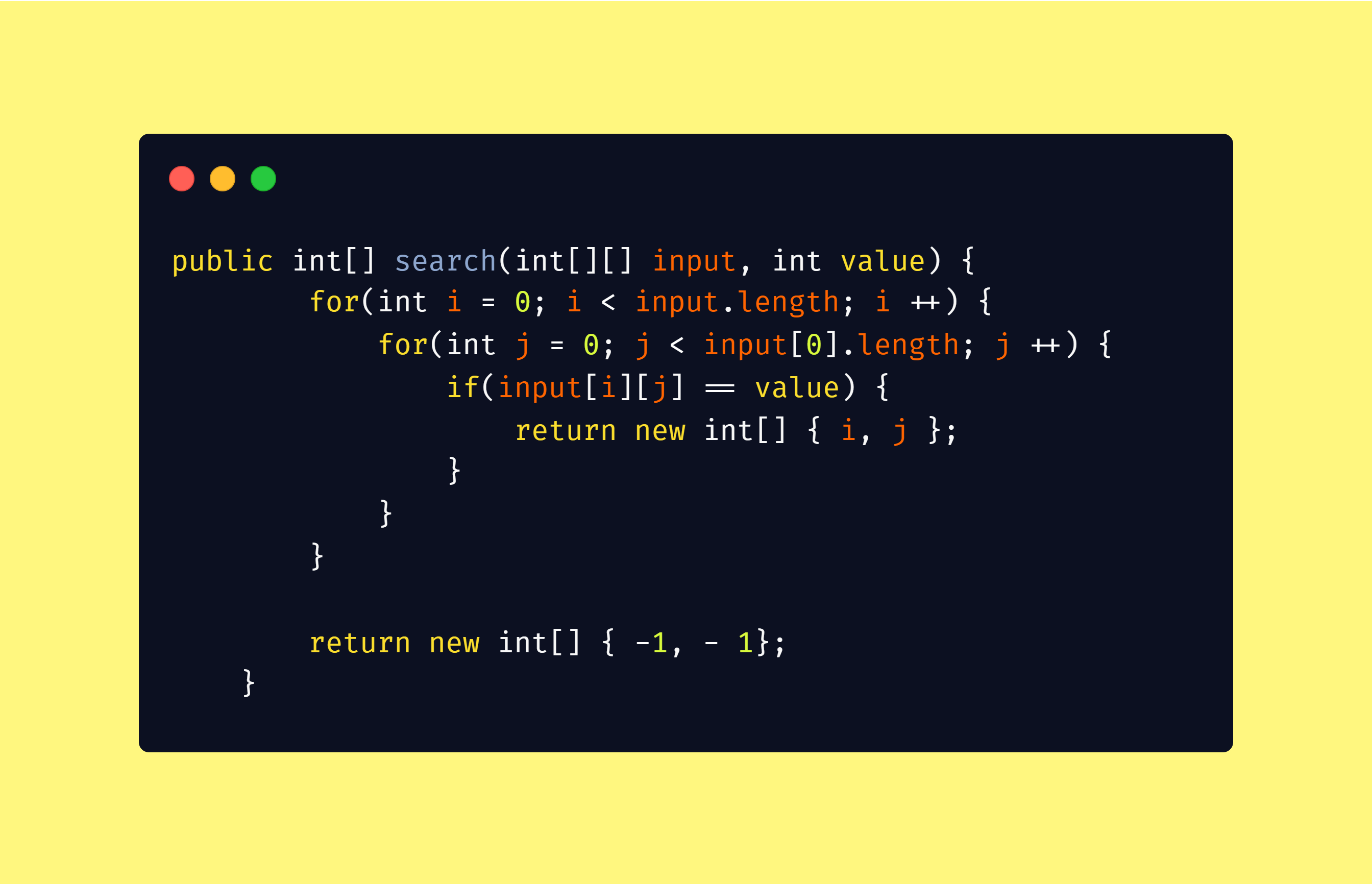1668503169

# What Is A* Search Algorithm?

In this Algorithm article, let's learn about What Is A* Search Algorithm?. Intelligence is the strength of the human species; we have used it to improve our lives. Then, we created the concept of artificial intelligence to amplify human intelligence and to develop and flourish civilizations like never before. A* Search Algorithm is one such algorithm that has been developed to help us. In this blog, we will learn more about what the A* algorithm in artificial intelligence means, the steps involved in the A* search algorithm in artificial intelligence, its implementation in Python, and more.

AI helps us solve problems of various complexities. Computational problems like path search problems can be solved using AI. Search problems where you need to find a path from one point to another, say, point A to point B. Sometimes you need to solve it by mapping those problems to graphs, where nodes represent all the possible outcomes. A* algorithm comes up as an answer to these problems.

Created as part of the Shakey project aimed to build a mobile robot that has artificial intelligence to plan its actions, A* was initially designed as a general graph traversal algorithm. It is widely used in solving pathfinding problems in video games.  Because of its flexibility and versatility, it can be used in a wide range of contexts. A* is formulated with weighted graphs, which means it can find the best path involving the smallest cost in terms of distance and time. This makes A* algorithm in artificial intelligence an informed search algorithm for best-first search. Let us have a detailed look into the various aspects of A*.

## What is A* Search Algorithm?

A* search algorithm is an algorithm that separates it from other traversal techniques. This makes A* smart and pushes it much ahead of conventional algorithms.

Let’s try to understand Basic AI Concepts and comprehend how does A* algorithm work. Imagine a huge maze that is too big that it takes hours to reach the endpoint manually. Once you complete it on foot, you need to go for another one. This implies that you would end up investing a lot of time and effort to find the possible paths in this maze. Now, you want to make it less time-consuming. To make it easier, we will consider this maze as a search problem and will try to apply it to other possible mazes we might encounter in due course, provided they follow the same structure and rules.

As the first step to converting this maze into a search problem, we need to define these six things.

1. A set of prospective states we might be in
2. A beginning and end state
3. A way to decide if we’ve reached the endpoint
4. A set of actions in case of possible direction/path changes
5. A function that advises us about the result of an action
6. A set of costs incurring in different states/paths of movement

To solve the problem, we need to map the intersections to the nodes (denoted by the red dots) and all the possible ways we can make movements towards the edges (denoted by the blue lines).
A denotes the starting point, and B denotes the endpoint. We define the starting and endpoints at nodes A and B, respectively.
If we use an uninformed search algorithm, it would be like finding a path that is blind, while an informed algorithm for a search problem would take the path that brings you closer to your destination. For instance, consider Rubik’s cube; it has many prospective states that you can be in, making the solution very difficult. This calls for the use of a guided search algorithm to find a solution. This explains the importance of A*.
Unlike other algorithms, A* decides to take up a step only if it is convincingly sensible and reasonable as per its functions. This means it never considers any non-optimal steps. This is why A* is a popular choice for AI systems that replicate the real world – like video games and machine learning.

## A* Search Algorithm Steps

Step 1: Add the beginning node to the open list
Step 2: Repeat the following step

In the open list, find the square with the lowest F cost, which denotes the current square. Now we move to the closed square.

Consider 8 squares adjacent to the current square and Ignore it if it is on the closed list or if it is not workable. Do the following if it is workable.

Check if it is on the open list; if not, add it. You need to make the current square as this square’s a parent. You will now record the different costs of the square, like the F, G, and H costs.

If it is on the open list, use G cost to measure the better path. The lower the G cost, the better the path. If this path is better, make the current square as the parent square. Now you need to recalculate the other scores – the G and F scores of this square.

You’ll stop:

If you find the path, you need to check the closed list and add the target square to it.

There is no path if the open list is empty and you cannot find the target square.

Step 3. Now you can save the path and work backward, starting from the target square, going to the parent square from each square you go, till it takes you to the starting square. You’ve found your path now.

## Why is A* Search Algorithm Preferred?

It’s easy to give movement to objects. But pathfinding is not simple. It is a complex exercise. The following situation explains it.

The task is to take the unit you see at the bottom of the diagram to the top of it. You can see that nothing indicates that the object should not take the path denoted with pink lines. So it chooses to move that way. As and when it reaches the top, it has to change its direction because of the ‘U’ shaped obstacle. Then it changes direction and goes around the obstacle to reach the top. In contrast to this, A* would have scanned the area above the object and found a short path (denoted with blue lines). Thus, pathfinder algorithms like A* help you plan things rather than waiting until you discover the problem. They act proactively rather than reacting to a situation. The disadvantage is that it is a bit slower than the other algorithms. You can use a combination of both to achieve better results – pathfinding algorithms give a bigger picture and long paths with obstacles that change slowly, and movement algorithms for a local picture and short paths with obstacles that change faster.

Read how artificial intelligence will create more jobs by 2025.

## A* Search Algorithm and Its Basic Concepts

A* algorithm works based on heuristic methods, and this helps achieve optimality. A* is a different form of the best-first algorithm. Optimality empowers an algorithm to find the best possible solution to a problem. Such algorithms also offer completeness; if there is any solution possible to an existing problem, the algorithm will definitely find it.

When A* enters into a problem, firstly, it calculates the cost to travel to the neighboring nodes and chooses the node with the lowest cost. If The f(n) denotes the cost, A* chooses the node with the lowest f(n) value. Here ‘n’ denotes the neighboring nodes. The calculation of the value can be done as shown below:

f(n)=g(n)+h(n)f(n)=g(n)+h(n)
g(n) = shows the shortest path’s value from the starting node to node n
h(n) = The heuristic approximation of the value of the node

The heuristic value has an important role in the efficiency of the A* algorithm. To find the best solution, you might have to use different heuristic functions according to the type of the problem. However, the creation of these functions is a difficult task, and this is the basic problem we face in AI.

## What is a Heuristic Function?A heuristic is simply called a heuristic function that helps rank the alternatives given in a search algorithm at each of its steps. It can either produce a result on its own or work in conjugation with a given algorithm to create a result. Essentially, a heuristic function helps algorithms to make the best decision faster and more efficiently. This ranking is based on the best available information and helps the algorithm decide the best possible branch to follow. Admissibility and consistency are the two fundamental properties of a heuristic function.

## Admissibility of the Heuristic Function

A heuristic function is admissible if it can effectively estimate the real distance between a node ‘n’ and the end node. It never overestimates; if it ever does, it will be denoted by ‘d’, which also denotes the accuracy of the solution.

## Consistency of the Heuristic Function

A heuristic function is consistent if the estimate of a given heuristic function turns out to be equal to or less than the distance between the goal (n) and a neighbor and the cost calculated to reach that neighbor.

A* is indeed a very powerful algorithm used to increase the performance of artificial intelligence. It is one of the most popular search algorithms in AI. The sky is the limit when it comes to the potential of this algorithm. However, the efficiency of an A* algorithm highly depends on the quality of its heuristic function. Wonder why this algorithm is preferred and used in many software systems? There is no single facet of AI where the A*algorithm has not found its application. From search optimization to games, robotics, and machine learning, the A* algorithm is an inevitable part of a smart program.

## Implementation with Python

In this section, we are going to find out how the A* search algorithm can be used to find the most cost-effective path in a graph. Consider the following graph below.The numbers written on edges represent the distance between the nodes, while the numbers written on nodes represent the heuristic values. Let us find the most cost-effective path to reach from start state A to final state G using the A* Algorithm.

Let’s start with node A. Since A is a starting node, therefore, the value of g(x) for A is zero, and from the graph, we get the heuristic value of A is 11, therefore

g(x) + h(x) = f(x) 0+ 11 =11 Thus for A, we can write A=11 Now from A, we can go to point B or point E, so we compute f(x) for each of them A → B = 2 + 6 = 8 A → E = 3 + 6 = 9Since the cost for  A → B is less, we move forward with this path and compute the f(x) for the children nodes of B Since there is no path between C and G, the heuristic cost is set to infinity or a very high value A → B → C = (2 + 1) + 99= 102 A → B → G = (2 + 9 ) + 0 = 11 Here the path A → B → G has the least cost but it is still more than the cost of A → E, thus we explore this path further A → E → D = (3 + 6) + 1 = 10 Comparing the cost of A → E → D with all the paths we got so far and as this cost is least of all we move forward with this path. And compute the f(x) for the children of D A → E → D → G = (3 + 6 + 1) +0 =10 Now comparing all the paths that lead us to the goal, we conclude that A → E → D → G is the most cost-effective path to get from A to G.Next, we write a program in Python that can find the most cost-effective path by using the a-star algorithm.

First, we create two sets, viz- open and close. The open contains the nodes that have been visited, but their neighbors are yet to be explored. On the other hand, close contains nodes that, along with their neighbors, have been visited.

Output:

Path Found: [ 'A','E','D','G']

## FAQs Related to A* Search Algorithm

How does the A * algorithm work?

A* Algorithm works by vertices in the graph, which start with the object’s starting point and then repeatedly examines the next unexamined vertex, adding its vertices to the set of vertices that will be examined.

What is the difference between the A* and AO* algorithm?

An A* is an OR graph algorithm used to find a single solution, while AO* Algorithm is an AND-OR graph algorithm used to find many solutions by ANDing over more than one branch.

Why is the A* algorithm popular?

A* Algorithm is popular because it is a technique that is used for finding path and graph traversals. Many web-based maps and games use this algorithm.

Is A* better than Dijkstra?

A* is usually considered better than Dijkstra as it performs informed and not uninformed searches. It expands more promising vertices.

Does Google Maps use the A* algorithm?

No. Google Maps uses the Dijkstra algorithm.

Why is A* optimal?

A* Algorithms are optimal. It relies on an open and closed list to find a path that is optimal and complete towards the goal.

How overestimation is handled in the A* algorithm?

Overestimation happens when the estimate of the heuristic is more than the actual cost of the final path.

Original article source at: https://www.mygreatlearning.com

## Buddha Community1603767600

## Search Algorithms

Today, let us touch base on some fundamental concepts like search algorithms.

In simple terms, **searching **is a process of looking up a particular data record in the database or in the collection of items. A search typically answers as true or false whether the particular data in which we are referring is found or not and perform the next action accordingly.

Commonly used algorithms for search are:

• Linear search
• Binary search
• Interpolation search

Let us understand them in detail with some example

## Linear Search Algorithm

Linear Search Algorithm is the simplest and easiest form of the search algorithm. In this algorithm, a sequential search is made over all the items one by one to search for the targeted item. Each item is checked in sequence until the match is found. If the match is found, the searched item is returned otherwise the search continues till the end.

To make it easy to understand, let us see understand linear search using a flow diagramLinear Search — Data Flow

### Points to note:

• Does not need sorted array list
• Performs equality comparisons
• The time complexity is O(n)
• Time taken to search elements keeps increasing as the number of elements is increased.

## Binary Search Algorithm

In _Binary search algorithm, _begins with an interval covering the whole array and diving it in half. If the value of the search key is less than the item in the middle of the interval, narrow the interval to the lower half. Otherwise narrow it to the upper half. Repeatedly check until the value is found or the interval is empty.

To make it easy to understand, let us see understand binary search using flow diagram and example as below.Binary Search — Data Flow

### Points to note:

• The array needs to be sorted
• Performs ordering comparisons
• Time complexity to O(log n).
• Search is done to either half of the given list, thus cut down your search to half time

#sorting-algorithms #algorithms #data-structures #search-and-sort #searching-algorithm1637911366

## Create a Product Filter and Search Using HTML, CSS & Javascript

Create a product filter that filters the products based on their categories using html, css & javascript . It also comes along with a search bar that searches the products based

### Source Code:

HTML file

``````<!DOCTYPE html>
<html lang="en">
<meta name="viewport" content="width=device-width, initial-scale=1.0" />
<title>Product Filter And Search</title>
rel="stylesheet"
/>
<!-- Stylesheet -->
<body>
<div class="wrapper">
<div id="search-container">
<input
type="search"
id="search-input"
placeholder="Search product name here.."
/>
<button id="search">Search</button>
</div>
<div id="buttons">
<button class="button-value" onclick="filterProduct('all')">All</button>
<button class="button-value" onclick="filterProduct('Topwear')">
Topwear
</button>
<button class="button-value" onclick="filterProduct('Bottomwear')">
Bottomwear
</button>
<button class="button-value" onclick="filterProduct('Jacket')">
Jacket
</button>
<button class="button-value" onclick="filterProduct('Watch')">
Watch
</button>
</div>
<div id="products"></div>
</div>
<!-- Script -->
<script src="script.js"></script>
</body>
</html>``````

CSS file

``````* {
margin: 0;
box-sizing: border-box;
border: none;
outline: none;
font-family: "Poppins", sans-serif;
}
body {
background-color: #f5f8ff;
}
.wrapper {
width: 95%;
margin: 0 auto;
}
#search-container {
margin: 1em 0;
}
#search-container input {
background-color: transparent;
width: 40%;
border-bottom: 2px solid #110f29;
}
#search-container input:focus {
border-bottom-color: #6759ff;
}
#search-container button {
margin-left: 1em;
background-color: #6759ff;
color: #ffffff;
margin-top: 0.5em;
}
.button-value {
border: 2px solid #6759ff;
background-color: transparent;
color: #6759ff;
cursor: pointer;
}
.active {
background-color: #6759ff;
color: #ffffff;
}
#products {
display: grid;
grid-template-columns: auto auto auto;
grid-column-gap: 1.5em;
}
.card {
background-color: #ffffff;
max-width: 18em;
margin-top: 1em;
box-shadow: 1em 2em 2.5em rgba(1, 2, 68, 0.08);
}
.image-container {
text-align: center;
}
img {
max-width: 100%;
object-fit: contain;
height: 15em;
}
.container {
color: #110f29;
}
.container h5 {
font-weight: 500;
}
.hide {
display: none;
}
@media screen and (max-width: 720px) {
img {
max-width: 100%;
object-fit: contain;
height: 10em;
}
.card {
max-width: 10em;
margin-top: 1em;
}
#products {
grid-template-columns: auto auto;
grid-column-gap: 1em;
}
}``````

JavaScript file

``````let products = {
data: [
{
productName: "Regular White T-Shirt",
category: "Topwear",
price: "30",
image: "white-tshirt.jpg",
},
{
productName: "Beige Short Skirt",
category: "Bottomwear",
price: "49",
image: "short-skirt.jpg",
},
{
productName: "Sporty SmartWatch",
category: "Watch",
price: "99",
image: "sporty-smartwatch.jpg",
},
{
productName: "Basic Knitted Top",
category: "Topwear",
price: "29",
image: "knitted-top.jpg",
},
{
productName: "Black Leather Jacket",
category: "Jacket",
price: "129",
image: "black-leather-jacket.jpg",
},
{
productName: "Stylish Pink Trousers",
category: "Bottomwear",
price: "89",
image: "pink-trousers.jpg",
},
{
productName: "Brown Men's Jacket",
category: "Jacket",
price: "189",
image: "brown-jacket.jpg",
},
{
productName: "Comfy Gray Pants",
category: "Bottomwear",
price: "49",
image: "comfy-gray-pants.jpg",
},
],
};

for (let i of products.data) {
//Create Card
let card = document.createElement("div");
//Card should have category and should stay hidden initially
//image div
let imgContainer = document.createElement("div");
//img tag
let image = document.createElement("img");
image.setAttribute("src", i.image);
imgContainer.appendChild(image);
card.appendChild(imgContainer);
//container
let container = document.createElement("div");
//product name
let name = document.createElement("h5");
name.innerText = i.productName.toUpperCase();
container.appendChild(name);
//price
let price = document.createElement("h6");
price.innerText = "\$" + i.price;
container.appendChild(price);

card.appendChild(container);
document.getElementById("products").appendChild(card);
}

//parameter passed from button (Parameter same as category)
function filterProduct(value) {
//Button class code
let buttons = document.querySelectorAll(".button-value");
buttons.forEach((button) => {
//check if value equals innerText
if (value.toUpperCase() == button.innerText.toUpperCase()) {
} else {
button.classList.remove("active");
}
});

//select all cards
let elements = document.querySelectorAll(".card");
//loop through all cards
elements.forEach((element) => {
//display all cards on 'all' button click
if (value == "all") {
element.classList.remove("hide");
} else {
//Check if element contains category class
if (element.classList.contains(value)) {
//display element based on category
element.classList.remove("hide");
} else {
//hide other elements
}
}
});
}

//Search button click
//initializations
let searchInput = document.getElementById("search-input").value;
let elements = document.querySelectorAll(".product-name");
let cards = document.querySelectorAll(".card");

//loop through all elements
elements.forEach((element, index) => {
//check if text includes the search value
if (element.innerText.includes(searchInput.toUpperCase())) {
//display matching card
cards[index].classList.remove("hide");
} else {
//hide others
}
});
});

filterProduct("all");
};``````1596737760

# Statement

We have to search for a value **x **in a sorted matrix M. If **exists, then return its coordinates **(i, j), else return (-1, -1).Let us consider the above matrix as an example. In this example, we are going to search for the value 12. Since 12 is present in the matrix, the algorithm should return its coordinates (2, 1)

# Simple Approach

A simple approach is to traverse all the values in the matrix and check if it is equal to 12.The worst case time complexity of the above algorithm will be

_O(n x m) = O(n²) __when _n = m

# Efficient Approach

The above algorithm behaves worse for large values of n and m. Let us look into the efficient algorithm now.

## Algorithm

``````1\. Start from Top Right position (0, m - 1) in the matrix M
2\. If the value is equal to x return (0, m - 1)
3\. Move one row down if the current value is less than x
4\. Move one column left if the current value is greater than x
``````

Let us apply this algorithm into our matrix M. We are going to search for the value 12 in M

#data-structures #search-sorted-matrix #2d-binary-search #algorithms #matrix-search #algorithms1597045745

## Searching Algorithms in Java

Searching Algorithms are designed to check for an element or retrieve an element from any data structure where it is stored. Based on the type of search operation, these algorithms are generally classified into two categories:

1. Sequential Search: In this, the list or array is traversed sequentially and every element is checked. For Example: Linear Search.
2. Interval Search: These algorithms are specifically designed for searching in sorted data-structures. These type of searching algorithms are much more efficient than Linear Search as they repeatedly target the center of the search structure and divide the search space in half. For Example: Binary Search.

Linear Search: The idea is to traverse the given array arr[] and find the index at which the element is present. Below are the steps:

• Let the element to be search be x.
• Start from the leftmost element of arr[] and one by one compare x with each element of arr[].
• If x matches with an element then return that index.
• If x doesn’t match with any of elements then return -1.

#java #searching #algorithms-searching #binary search1598518020

## Let Us Understand Searching Algorithms

When searching for data, the difference between a fast application and a slower one lies in the accurate use of search algorithm. Searching algorithms is a basic, fundamental step in computing done via step-by-step method to locate a specific data among a collection of data.

All search algorithms make use of a search key in order to complete the procedure. And they are expected to return a success or a failure status ( in boolean true or false value).

In computer science, there are various type of search algorithms available and the way they are used decides the performance and efficiency of the data available( the manner in which the data is being used).

What is a Search Algorithm?

According to Wikipedia- Search algorithm is-

Any algorithm which solves the search problem, namely, to retrieve information stored within some data structure, or calculated in the search space of a problem domain, either with discrete or continuous values.

Searching Algorithms are designed to check or retrieve an element from any data structure where it is being stored.

They search for a target (key) in the search space, like-

1. All students in the class.
2. All numbers in a given list.

#data structure #algorithm skills #algorithms #searching algorithms #big data